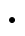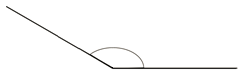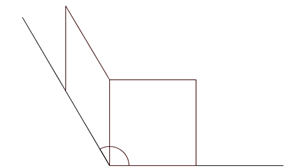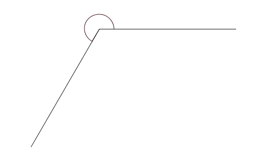# Shapes and Angles

Lesson 11

Math

Unit 8

Lesson 11 of 18

## Objective

Decompose angles using pattern blocks.

## Common Core Standards

### Core Standards

• 4.MD.C.7 — Recognize angle measure as additive. When an angle is decomposed into non-overlapping parts, the angle measure of the whole is the sum of the angle measures of the parts. Solve addition and subtraction problems to find unknown angles on a diagram in real world and mathematical problems, e.g., by using an equation with a symbol for the unknown angle measure.

## Criteria for Success

1. Understand that the measures of all of the angles at a point with no gaps or overlaps add up to 360°, and use that understanding to find the measures of pattern blocks’ angles.
2. Find the measure of various angles by filling the angle with pattern blocks and adding the angle measures of those pattern blocks together.
3. Understand that angle measure is additive (i.e., when an angle is decomposed into non-overlapping parts, the angle measure of the whole is the sum of the angle measures of the parts) (MP.2).

## Tips for Teachers

• The teacher may want to display pattern blocks so that they are visible to all students, which can be done by placing them on an overhead projector, by sticking magnets on a set of pattern blocks to use on their white/chalkboard, or by using an online applet (like this one from NCTM Illuminations).

### Lesson Materials

• pattern blocks (4 squares, 6 triangles, 3 hexagons, 6 blue rhombuses, 12 tan rhombuses, per student or small group) — These are also needed for the Problem Set.
• 180° Protractor (1 per student)
Fishtank Plus

Unlock features to optimize your prep time, plan engaging lessons, and monitor student progress.

### Problem 1

1. Place square pattern blocks around the point below so that their corners meet at the point. Fit them like puzzle pieces. How many angles meet at this central point? What is the measure of each of them? Write a number sentence to represent this relationship.1. Go through the same process as above with triangle pattern blocks.

#### Guiding Questions

Create a free account or sign in to access the Guiding Questions for this Anchor Problem.

#### References

EngageNY Mathematics Grade 4 Mathematics > Module 4 > Topic C > Lesson 9Concept Development, Problem 1

Grade 4 Mathematics > Module 4 > Topic C > Lesson 9 of the New York State Common Core Mathematics Curriculum from EngageNY and Great Minds. © 2015 Great Minds. Licensed by EngageNY of the New York State Education Department under the CC BY-NC-SA 3.0 US license. Accessed Dec. 2, 2016, 5:15 p.m..

Modified by Fishtank Learning, Inc.

### Problem 2

Using pattern blocks and their known measure, find the measure of the angle indicated by the arc.#### Guiding Questions

Create a free account or sign in to access the Guiding Questions for this Anchor Problem.

#### References

EngageNY Mathematics Grade 4 Mathematics > Module 4 > Topic C > Lesson 9Concept Development Problem 3

Grade 4 Mathematics > Module 4 > Topic C > Lesson 9 of the New York State Common Core Mathematics Curriculum from EngageNY and Great Minds. © 2015 Great Minds. Licensed by EngageNY of the New York State Education Department under the CC BY-NC-SA 3.0 US license. Accessed Dec. 2, 2016, 5:15 p.m..

Modified by Fishtank Learning, Inc.

## Problem Set & Homework

Answer keys for Problem Sets and Homework are available with a Fishtank Plus subscription.

### Discussion of Problem Set

• What are the measures for the acute and obtuse angles of the tan rhombus? What did you discover when you fit the acute angles around a vertex?
• How are the different angles in the pattern blocks related?
• What was the measure of $${\angle HIJ}$$? $${\angle L}$$? $${\angle O}$$? $${\angle R}$$? How did you find the angle measures? What combination of blocks did you use? How did your method compare with your neighbor's?
• What did you learn about adding angles?
• How can you verify an angle's measure?

### Problem 1

Find the measurements of the angles indicated by the arcs.

 Pattern blocks Angle measure Addition sentence### Problem 2

Use two or more pattern blocks to figure out the measurements of the angles indicated by the arcs.

 Pattern blocks Angle measure Addition sentence### Student Response

Fishtank Plus Content

Word Problems and Fluency Acitivities

Access daily word problem practice and our content-aligned fluency activities created to help students strengthen their application and fluency skills.Lesson 10

Lesson 12

## Lesson Map

Topic A: Lines and Angles

Topic B: Measures of Angles

Topic C: Measures of Adjacent Angles

Topic D: Shapes and Lines of Symmetry